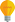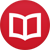# Arithmetics in which you can divide by zero

by Jakub MarianTip: See my list of theMost Common Mistakes in English. It will teach you how to avoid mis­takes with com­mas, pre­pos­i­tions, ir­reg­u­lar verbs, and much more.

When I was still in primary school, I was fascinated by things you “can’t do” in mathematics and developed a “theory” of division by zero. I didn’t really see it at the time, but what I did was that I defined a new set which contained (a copy of) $ℝ$ as a subset and changed the rules for multiplication by zero so that it was compatible with my proposed division by zero. It turned out it kind of worked, but there were some serious limitations.

I did the following: Instead of mere numbers, our objects are pairs $(x,n)$ where $x ∈ ℝ \setminus \{0\}$ and $n ∈ ℤ$. The $n$ in $(x,n)$ is called the order of the zero. Instead of $(x,0)$ we will write simply $x$, with the exception of $0$ which means $(1,1)$ (“one zero” of the first order). Addition is defined as

$$(x,m)+(y,n) = \begin{cases} (x+y,m) & \text{if } m=n, x≠-y \\ (1,1) = 0 & \text{if } m=n, x=-y \\ (x,m) & \text{if }m < n \\ (y,n) & \text{if }m > n\end{cases}$$

The idea behind this is that $(x,n)$ is supposed to represent $x⋅0^n$. When computing $x⋅0^n + y⋅0^m$, if $n > m$, $0^n$ is a zero of a greater order than $0^m$, i.e. it is infinitely many times “smaller” and can be ignored.

Since $(x,n)$ is supposed to represent $x⋅0^n$, $x⋅0^n⋅y⋅0^m$ should be $xy⋅0^{n+m}$, so multiplication is defined as

$$(x,m)⋅(y,n) = (xy,m+n)\,.$$

In particular, $0^n = (1,1)^n = (1,n)$, so, from now on, we will write $x⋅0^n$ instead of $(x,n)$. Division is defined as

$$÷{x⋅0^n}{y⋅0^m} = ÷{x}{y}⋅0^{n-m}\,.$$

The last symbol we will define is $∞ = 0^{-1} = (1,-1)$. Using the definition of division above and the definition of $∞$, we get that

$$÷{1}{0} = ∞,\quad ÷{1}{∞} = 0\,.$$

Even more interestingly, we get

$$∞⋅0 = 1\,.$$

So the arithmetics is quite consistent. In general, it’s true that

$$÷{x}{0}⋅0 = x⋅∞⋅0 = x⋅1 = x\,,$$

and similarly

$$÷{x}{∞}⋅∞ = x\,.$$

## Did we get a good theory for division by zero?

What did we have to sacrifice to have that? The first oddity is that addition is no longer in general associative, for example:

$$(∞+(-∞))+5 = 0+5 = 5\quad\text{vs.}\quad ∞+((-∞)+5) = ∞+(-∞) = 0\,.$$

However, a more serious problem is that distributivity breaks down when simplifying expressions containing a difference of the form $x-x$:

$$3⋅(x-x) = 3⋅0 ≠ 0 = 3x -3x\,.$$

The problem with non-associativity in addition could seemingly be fixed by not forgetting the lower order terms, but in fact it would become even worse; for example $-2+2+2$ is associative with forgetting, but if we didn’t forget lower order terms, we’d get $(-2+2)+2 = 0+2 ≠ 2 = -2+(2+2)$, not to mention that the fact that $0+2 ≠ 2$ is itself somewhat unsatisfactory.

The problem with distributivity cannot be solved in principle, unless $x-x$ is an “absolute zero”, i.e. something that multiplied with anything gives the same zero, as demonstrated above. However, as soon as there is an “absolute zero” in the theory, there is no way to define $÷{1}{0}$. The only other way to make distributivity work would be to define $x-x=x⋅0$ (this follows directly from the equation $x⋅0$ $=$ $x(1-1)$ $=$ $x-x$), but this would break commutativity because $-x+x = (-x)⋅0$ whereas $x-x = x⋅0$.

The moral of the story is: We can define an arithmetics in which division by zero is possible. However, in order to do that, we sacrifice some of the most fundamental arithmetical rules we are used to. This wouldn’t be a bad thing per se if we got something interesting in return. The problem is that I am not aware of any mathematical or real-life problem where this new structure would be more useful than the classical one.

By the way, I have written several educational ebooks. If you get a copy, you can learn new things and support this website at the same time—why don’t you check them out?